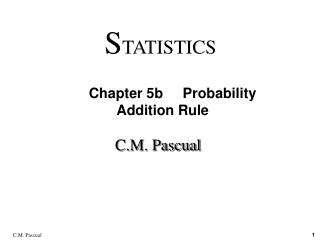DownloadDownload PresentationC.M. Pascual

# C.M. Pascual

Télécharger la présentation## C.M. Pascual

- - - - - - - - - - - - - - - - - - - - - - - - - - - E N D - - - - - - - - - - - - - - - - - - - - - - - - - - -
##### Presentation Transcript

1. STATISTICS Chapter 5b Probability Addition Rule C.M. Pascual

2. Compound Event Any event combining 2 or more simple    events Definition

3. Compound Event Any event combining 2 or more simple    events Notation P(A or B) = P (event A occurs or event B occurs or they both occur) Definition

4. General Rule When finding the probability that event A occurs or event B occurs, find the total number of ways A can occur and the number of ways B can occur, but find the total in such a way that no outcome is counted more than once. Compound Event

5. Formal Addition Rule P(A or B) = P(A) + P(B) - P(A and B) where P(A and B) denotes the probability that A and Bboth occur at the same time. Compound Event

6. Formal Addition Rule P(A or B) = P(A) + P(B) - P(A and B) where P(A and B) denotes the probability that A and Bboth occur at the same time. Intuitive Addition Rule To find P(A or B), find the sum of the number of ways event A can occur and the number of ways event B can occur, adding in such a way that every outcome iscounted only once. P(A or B) is equal to that sum, divided by the total number of outcomes. Compound Event

7. Events A and B are mutually exclusive if they cannot occur simultaneously. Definition

8. Events A and B are mutually exclusive if they cannot occur simultaneously. Definition Total Area = 1 P(A) P(B) P(A and B) Overlapping Events Figures 3-5

9. Events A and B are mutually exclusive if they cannot occur simultaneously. Definition Total Area = 1 Total Area = 1 P(A) P(B) P(A) P(B) P(A and B) Overlapping Events Non-overlapping Events Figures 3-5 and 3-6

10. Figure 5-7 Applying the Addition Rule P(A or B) Addition Rule Are A and B mutually exclusive ? Yes P(A or B) = P(A) + P(B) No P(A or B) = P(A)+ P(B) - P(A and B)

11. Find the probability of randomly selecting a man or a boy. Contingency Table Men Women Boys Girls Totals Survived 332 318 29 27 706 Died 1360 104 35 18 1517 Total 1692 422 64 56 2223

12. Find the probability of randomly selecting a man or a boy. Contingency Table Men Women Boys Girls Totals Survived 332 318 29 27 706 Died 1360 104 35 18 1517 Total 1692 422 64 56 2223

13. Find the probability of randomly selecting a man or a boy. P(man or boy) = 1692 + 64 = 1756 = 0.790 2223 2223 2223 Contingency Table Men Women Boys Girls Totals Survived 332 318 29 27 706 Died 1360 104 35 18 1517 Total 1692 422 64 56 2223

14. Find the probability of randomly selecting a man or a boy. P(man or boy) = 1692 + 64 = 1756 = 0.790 2223 2223 2223 Contingency Table Men Women Boys Girls Totals Survived 332 318 29 27 706 Died 1360 104 35 18 1517 Total 1692 422 64 56 2223 * Mutually Exclusive *

15. Find the probability of randomly selecting a man or someone who survived. Contingency Table Men Women Boys Girls Totals Survived 332 318 29 27 706 Died 1360 104 35 18 1517 Total 1692 422 64 56 2223

16. Find the probability of randomly selecting a man or someone who survived. Contingency Table Men Women Boys Girls Totals Survived 332 318 29 27 706 Died 1360 104 35 18 1517 Total 1692 422 64 56 2223

17. Find the probability of randomly selecting a man or someone who survived. P(man or survivor) = 1692 + 706 - 332 = 1756 2223 2223 2223 2223 Contingency Table Men Women Boys Girls Totals Survived 332 318 29 27 706 Died 1360 104 35 18 1517 Total 1692 422 64 56 2223 = 0.929

18. Find the probability of randomly selecting a man or someone who survived. P(man or survivor) = 1692 + 706 - 332 = 1756 2223 2223 2223 2223 Contingency Table Men Women Boys Girls Totals Survived 332 318 29 27 706 Died 1360 104 35 18 1517 Total 1692 422 64 56 2223 = 0.929 * NOT Mutually Exclusive *

19. Complementary Events

20. P(A) and P(A) are mutually exclusive Complementary Events

21. P(A) and P(A) are mutually exclusive All simple events are either in A or A. Complementary Events

22. P(A) and P(A) are mutually exclusive All simple events are either in A or A. P(A) + P(A) = 1 Complementary Events

23. Rules of Complementary Events P(A) + P(A) = 1

24. Rules of Complementary Events P(A) + P(A) = 1 = 1 - P(A) P(A)

25. Rules of Complementary Events P(A) + P(A) = 1 = 1 - P(A) P(A) = 1 - P(A) P(A)

26. Figure 5-8 Venn Diagram for the Complement of Event A Total Area = 1 P (A) P (A) = 1 - P (A)